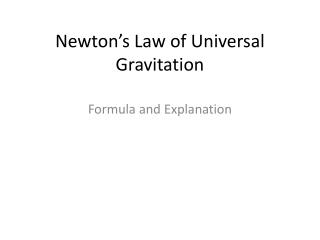DownloadDownload PresentationNewton’s Law of Universal Gravitation

# Newton’s Law of Universal Gravitation

Download Presentation## Newton’s Law of Universal Gravitation

- - - - - - - - - - - - - - - - - - - - - - - - - - - E N D - - - - - - - - - - - - - - - - - - - - - - - - - - -
##### Presentation Transcript

1. Newton’s Law of Universal Gravitation Formula and Explanation

2. Newton’s Law of Universal Gravitation • To calculate the force of gravitation • F = G m1m2 d2 • m1 and m2 = the mass of the objects in kgG = gravitational constant of 6.67 x 10 -11 N*m2/kg2d is the distance between the objects in mF = gravitational force between the 2 objects

3. Gravitational Force • If the value of the constant gravitational force G were to increase by a factor of 10, we would be crushed • If G were to decrease by a factor of 10, we would be able to jump over a tall building

4. Sample Problem 1 • Determine the force of gravitational attraction between the earth (m = 5.98 x 1024 kg) and a 70-kg physics student if the student is standing at sea level, a distance of 6.38 x 106 m from earth's center. • F = Gm1m2 = (6.67 x 10 -11 N*m2/kg2 )*(5.98 x 1024 kg )*(70kg) d2 (6.38 x 106m)2 • F = 686N

5. Sample Problem 2 • Determine the force of gravitational attraction between the earth (m = 5.98 x 1024 kg) and a 70-kg physics student if the student is in an airplane at 40000 feet above earth's surface. This would place the student a distance of 6.39 x 106 m from earth's center. • F =Gm1m2 = (6.67 x 10 -11 N*m2/kg2 )*(5.98 x 1024 kg )*(70kg) d2 (6.39 x 106m)2 • F = 684 N